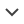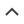### Data Platform

#### Weather Data

https://doi.org/10.25832/weather_data/2020-09-16
Package version
Description Hourly geographically aggregated weather data for Europe
Notes This data package contains radiation and temperature data, at hourly resolution, for Europe, aggregated by Renewables.ninja from the NASA MERRA-2 reanalysis. It covers the European countries using a population-weighted mean across all MERRA-2 grid cells within the given country.
Last changes Include radiation and temperature data up to 2019
Geographical scope Europe
Documentation Documentation and script (view on GitHub)
opsd-weather_data-2020-09-16.zip (74 MB)

Individual data files (csv, xlsx)
weather_data.csv (223 MB) Alternative file formatsFilterDatabase (SQLite)
weather_data.sqlite (223 MB)
datapackage.json
Input data Not provided
Sources NASA | Renewables.ninja
Report issue Report an issue on Github
Contact Stefan Pfenninger | Iain Staffell
Subscribe Get notified by email for updates
Attribution in Chicago author-date style should be given as follows: "Open Power System Data. 2020. Data Package Weather Data. Version 2020-09-16. https://doi.org/10.25832/weather_data/2020-09-16. (Primary data from various sources, for a complete list see URL)."
<- back to overview

### Field documentation

#### weather data.csv

Field Name Type (Format) Description
utc_timestamp datetime (fmt:%Y-%m-%dT%H%M%SZ) Start of time period in Coordinated Universal Time
AT_temperature number (float) temperature weather variable for AT in degrees C
AT_radiation_direct_horizontal number (float) radiation_direct_horizontal weather variable for AT in W/m2
AT_radiation_diffuse_horizontal number (float) radiation_diffuse_horizontal weather variable for AT in W/m2
BE_temperature number (float) temperature weather variable for BE in degrees C
BE_radiation_direct_horizontal number (float) radiation_direct_horizontal weather variable for BE in W/m2
BE_radiation_diffuse_horizontal number (float) radiation_diffuse_horizontal weather variable for BE in W/m2
BG_temperature number (float) temperature weather variable for BG in degrees C
BG_radiation_direct_horizontal number (float) radiation_direct_horizontal weather variable for BG in W/m2
BG_radiation_diffuse_horizontal number (float) radiation_diffuse_horizontal weather variable for BG in W/m2
CH_temperature number (float) temperature weather variable for CH in degrees C
CH_radiation_direct_horizontal number (float) radiation_direct_horizontal weather variable for CH in W/m2
CH_radiation_diffuse_horizontal number (float) radiation_diffuse_horizontal weather variable for CH in W/m2
CZ_temperature number (float) temperature weather variable for CZ in degrees C
CZ_radiation_direct_horizontal number (float) radiation_direct_horizontal weather variable for CZ in W/m2
CZ_radiation_diffuse_horizontal number (float) radiation_diffuse_horizontal weather variable for CZ in W/m2
DE_temperature number (float) temperature weather variable for DE in degrees C
DE_radiation_direct_horizontal number (float) radiation_direct_horizontal weather variable for DE in W/m2
DE_radiation_diffuse_horizontal number (float) radiation_diffuse_horizontal weather variable for DE in W/m2
DK_temperature number (float) temperature weather variable for DK in degrees C
DK_radiation_direct_horizontal number (float) radiation_direct_horizontal weather variable for DK in W/m2
DK_radiation_diffuse_horizontal number (float) radiation_diffuse_horizontal weather variable for DK in W/m2
EE_temperature number (float) temperature weather variable for EE in degrees C
EE_radiation_direct_horizontal number (float) radiation_direct_horizontal weather variable for EE in W/m2
EE_radiation_diffuse_horizontal number (float) radiation_diffuse_horizontal weather variable for EE in W/m2
ES_temperature number (float) temperature weather variable for ES in degrees C
ES_radiation_direct_horizontal number (float) radiation_direct_horizontal weather variable for ES in W/m2
ES_radiation_diffuse_horizontal number (float) radiation_diffuse_horizontal weather variable for ES in W/m2
FI_temperature number (float) temperature weather variable for FI in degrees C
FI_radiation_direct_horizontal number (float) radiation_direct_horizontal weather variable for FI in W/m2
FI_radiation_diffuse_horizontal number (float) radiation_diffuse_horizontal weather variable for FI in W/m2
FR_temperature number (float) temperature weather variable for FR in degrees C
FR_radiation_direct_horizontal number (float) radiation_direct_horizontal weather variable for FR in W/m2
FR_radiation_diffuse_horizontal number (float) radiation_diffuse_horizontal weather variable for FR in W/m2
GB_temperature number (float) temperature weather variable for GB in degrees C
GB_radiation_direct_horizontal number (float) radiation_direct_horizontal weather variable for GB in W/m2
GB_radiation_diffuse_horizontal number (float) radiation_diffuse_horizontal weather variable for GB in W/m2
GR_temperature number (float) temperature weather variable for GR in degrees C
GR_radiation_direct_horizontal number (float) radiation_direct_horizontal weather variable for GR in W/m2
GR_radiation_diffuse_horizontal number (float) radiation_diffuse_horizontal weather variable for GR in W/m2
HR_temperature number (float) temperature weather variable for HR in degrees C
HR_radiation_direct_horizontal number (float) radiation_direct_horizontal weather variable for HR in W/m2
HR_radiation_diffuse_horizontal number (float) radiation_diffuse_horizontal weather variable for HR in W/m2
HU_temperature number (float) temperature weather variable for HU in degrees C
HU_radiation_direct_horizontal number (float) radiation_direct_horizontal weather variable for HU in W/m2
HU_radiation_diffuse_horizontal number (float) radiation_diffuse_horizontal weather variable for HU in W/m2
IE_temperature number (float) temperature weather variable for IE in degrees C
IE_radiation_direct_horizontal number (float) radiation_direct_horizontal weather variable for IE in W/m2
IE_radiation_diffuse_horizontal number (float) radiation_diffuse_horizontal weather variable for IE in W/m2
IT_temperature number (float) temperature weather variable for IT in degrees C
IT_radiation_direct_horizontal number (float) radiation_direct_horizontal weather variable for IT in W/m2
IT_radiation_diffuse_horizontal number (float) radiation_diffuse_horizontal weather variable for IT in W/m2
LT_temperature number (float) temperature weather variable for LT in degrees C
LT_radiation_direct_horizontal number (float) radiation_direct_horizontal weather variable for LT in W/m2
LT_radiation_diffuse_horizontal number (float) radiation_diffuse_horizontal weather variable for LT in W/m2
LU_temperature number (float) temperature weather variable for LU in degrees C
LU_radiation_direct_horizontal number (float) radiation_direct_horizontal weather variable for LU in W/m2
LU_radiation_diffuse_horizontal number (float) radiation_diffuse_horizontal weather variable for LU in W/m2
LV_temperature number (float) temperature weather variable for LV in degrees C
LV_radiation_direct_horizontal number (float) radiation_direct_horizontal weather variable for LV in W/m2
LV_radiation_diffuse_horizontal number (float) radiation_diffuse_horizontal weather variable for LV in W/m2
NL_temperature number (float) temperature weather variable for NL in degrees C
NL_radiation_direct_horizontal number (float) radiation_direct_horizontal weather variable for NL in W/m2
NL_radiation_diffuse_horizontal number (float) radiation_diffuse_horizontal weather variable for NL in W/m2
NO_temperature number (float) temperature weather variable for NO in degrees C
NO_radiation_direct_horizontal number (float) radiation_direct_horizontal weather variable for NO in W/m2
NO_radiation_diffuse_horizontal number (float) radiation_diffuse_horizontal weather variable for NO in W/m2
PL_temperature number (float) temperature weather variable for PL in degrees C
PL_radiation_direct_horizontal number (float) radiation_direct_horizontal weather variable for PL in W/m2
PL_radiation_diffuse_horizontal number (float) radiation_diffuse_horizontal weather variable for PL in W/m2
PT_temperature number (float) temperature weather variable for PT in degrees C
PT_radiation_direct_horizontal number (float) radiation_direct_horizontal weather variable for PT in W/m2
PT_radiation_diffuse_horizontal number (float) radiation_diffuse_horizontal weather variable for PT in W/m2
RO_temperature number (float) temperature weather variable for RO in degrees C
RO_radiation_direct_horizontal number (float) radiation_direct_horizontal weather variable for RO in W/m2
RO_radiation_diffuse_horizontal number (float) radiation_diffuse_horizontal weather variable for RO in W/m2
SE_temperature number (float) temperature weather variable for SE in degrees C
SE_radiation_direct_horizontal number (float) radiation_direct_horizontal weather variable for SE in W/m2
SE_radiation_diffuse_horizontal number (float) radiation_diffuse_horizontal weather variable for SE in W/m2
SI_temperature number (float) temperature weather variable for SI in degrees C
SI_radiation_direct_horizontal number (float) radiation_direct_horizontal weather variable for SI in W/m2
SI_radiation_diffuse_horizontal number (float) radiation_diffuse_horizontal weather variable for SI in W/m2
SK_temperature number (float) temperature weather variable for SK in degrees C
SK_radiation_direct_horizontal number (float) radiation_direct_horizontal weather variable for SK in W/m2
SK_radiation_diffuse_horizontal number (float) radiation_diffuse_horizontal weather variable for SK in W/m2
<- back to overview